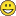# Convert point feature class to square polygon (points represent NE corner)

6142
9
04-20-2016 03:04 PM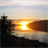New Contributor III

I have a point feature class in which each point represents the NE (Upper Left) corner of a 5' x 5' excavation unit across an archaeological site (the units are not contiguous).  I would like to generate a polygon feature of the 5' x 5' units (in other words, create the other 3 corners - LL, LR and UR and connect them with a closed line and convert to a polygon - (or directly create a polygon feature class if that's possible). Are there existing tools that I can use to do this, or a script that can be modified? I am not a programmer ;-(...I am using ArcGIS Desktop with an Advanced license.

Thanks so much!

Susan

9 RepliesbyMVP Esteemed Contributor

sorry... I think in code... there are options here , you are effectively producing a square buffer, except you are offsetting the focus point from the center to the NE corner ... sooo you will have to make some changes to the code.Regular Contributor II

there is so easy way to do that, but I want to now your spatial reference, and unitbyMVP Esteemed Contributor

Abdullah

all she needs to do is change the following lines, using a projected featureclass or shapefile.  Specify the width and height

```width = 100.0     # width of the output rectangle  in meters
height = 100.0   # height of the output rectangle
hX     =  50.0    # this can be +ve or -ve depending which corner you want to point to be focussed on
hY     = -50.0   # this is for Y  (50, -50) for hX and hY will place the origin point in the upper left```

# output change this obviously

`fc_out = r'D:\Xander\EPM\Datos pruebas\gdb\datos erase.gdb\rectangles3'`

and modify the values here

```def createRectangleFromPoint(pnt, width, height, hlf, sr):  # hlf is the correction to position the point
arrPnts = arcpy.Array()
pnt2 = arcpy.Point(pnt.X - width + hX, pnt.Y - height + hY)
pnt2 = arcpy.Point(pnt.X - width + hX, pnt.Y + height + hY)
pnt2 = arcpy.Point(pnt.X + width + hX, pnt.Y + height + hY)
pnt2 = arcpy.Point(pnt.X + width + hX, pnt.Y - height + hY)
pnt2 = arcpy.Point(pnt.X - width + hX, pnt.Y - height + hY)
return arcpy.Polygon(arrPnts, sr) ```Regular Contributor II

Yes,Dan you are right.

just there is an easy method using attribute table and toolbox without python.

I thought that maybe easier.New Contributor III

Hi there,

Thank you for the replies! I'm curious about your "easier" solution - I am using a custom coordinate (simply called Local Cartesian Coordinate System) with the following parameters, and the units are in feet:

Local Cartesian Coordinate System

Authority: Custom

Projection: Local

False_Easting: 0.0

False_Northing: 0.0

Scale_Factor: 1.0

Azimuth: 45.0

Longitude_Of_Center: -75.0

Latitude_Of_Center: 40.0

Linear Unit: Foot (0.3048)

Geographic Coordinate System: GCS_WGS_1984

Angular Unit: Degree (0.0174532925199433)

Prime Meridian: Greenwich (0.0)

Datum: D_WGS_1984

Spheroid: WGS_1984

Semimajor Axis: 6378137.0

Semiminor Axis: 6356752.314245179

Inverse Flattening: 298.257223563byMVP Esteemed Contributor

still looks geographic and the 'difficult' one, will get the spatial reference if it is defined and is indeed projected with units of feet, you only need to specify your buffer size  in feet.  You only run into difficulties when the coordinates are in decimal degrees and you want outputs in feet or meters.New Contributor III

Excellent. Thank you!Regular Contributor II

the  "easier" solution, in my opinion. that

1. use "Add XY Coordinates " for create x and y fields in the point feature class. Or create and Y fields manually , but make sure that your unit is feet (as your example).
2. create two fields too, for calculate the opposite corner (lower right).

you can calculate this using field calculator

• oppsite corner x = [POINT_X] + (5/12)    "that if you want create square polygon width 5 inches"
• oppsite corner y = [POINT_Y] - (5/12)    "that if you want create square polygon width 5 inches"

5/12 is variable you can change a value as you want with 2d of polygon.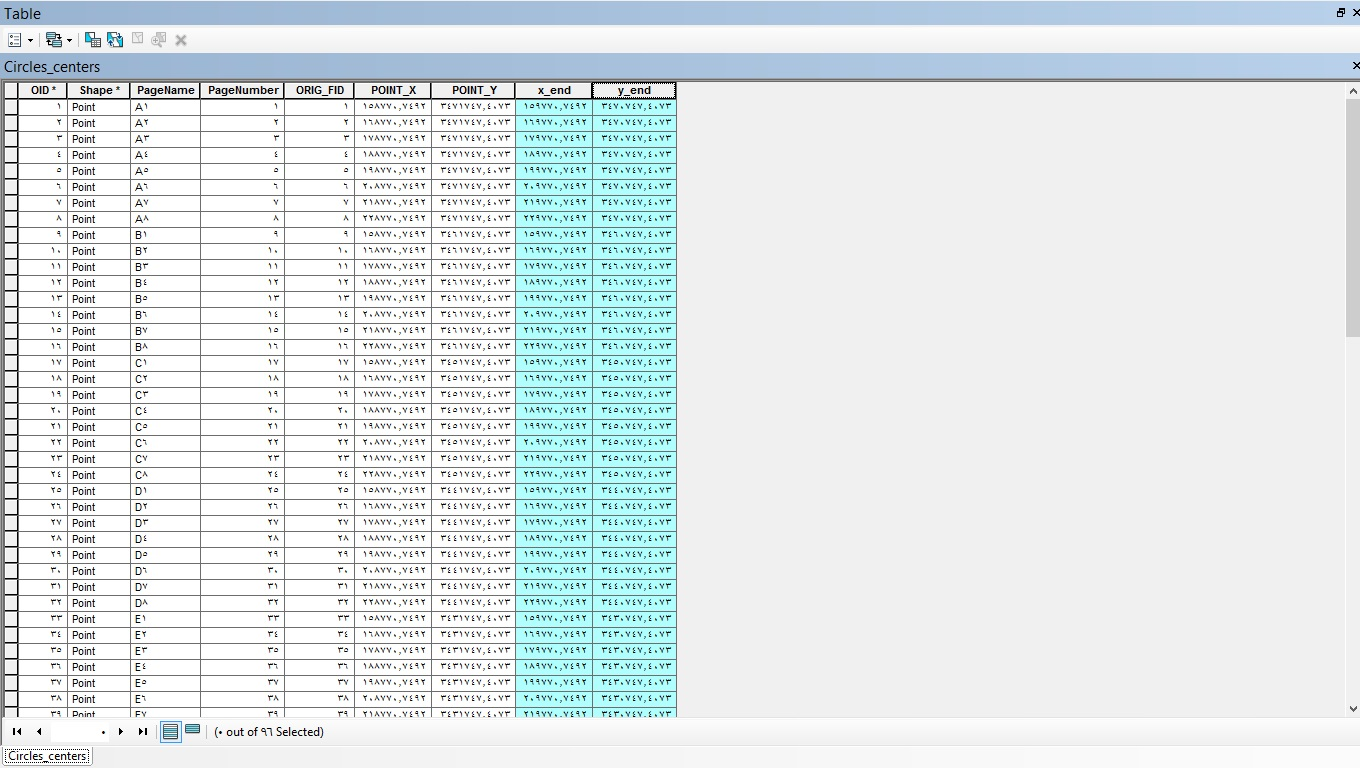3.  use " XY TO Line" tool and make start point the upper left coordinate , and end point lower right coordinate.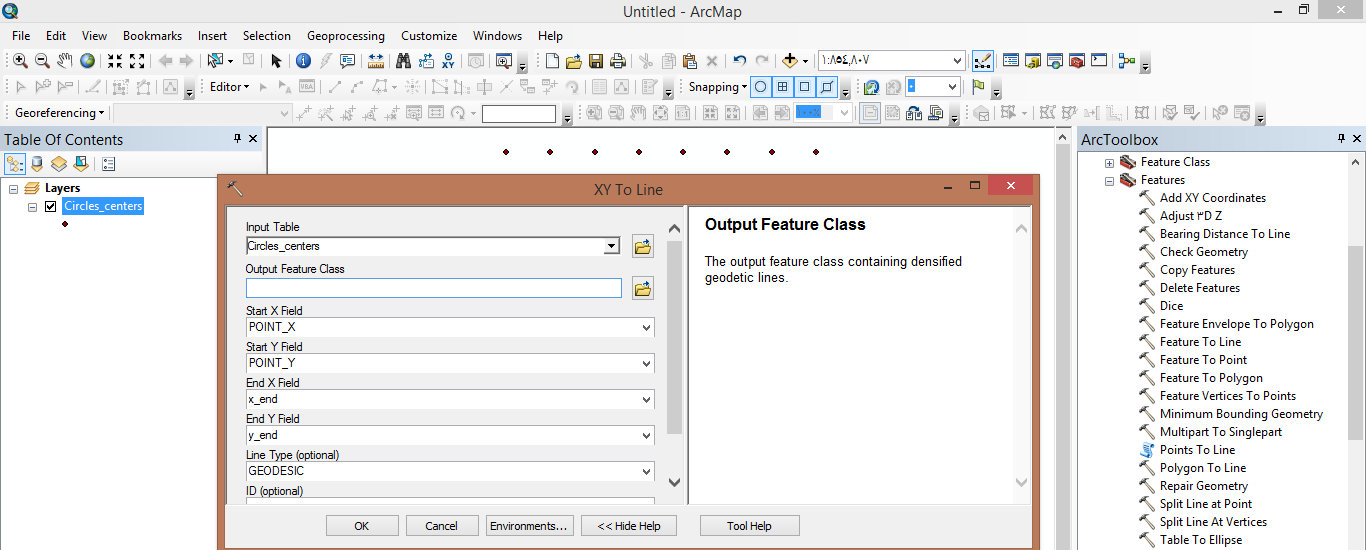4.  now you can get your polygons using "Feature Envelope To Polygon" and put the pervious line feature as input and check Create multipart features box . The output will be your polygon.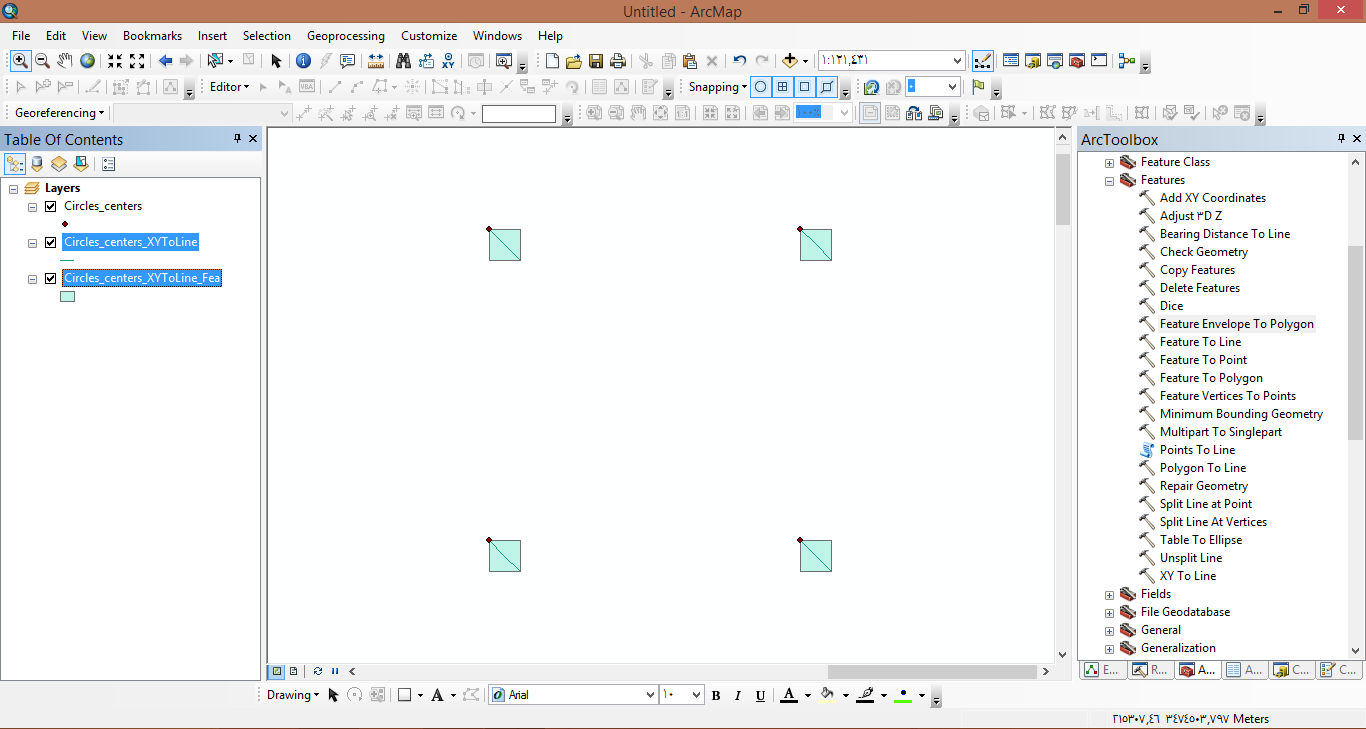thanks.New Contributor III

Again, thank you, very much - this was the solution I employed